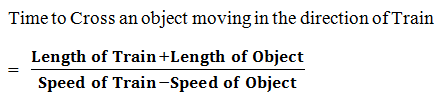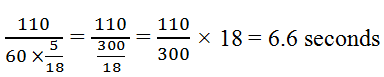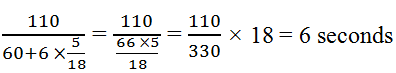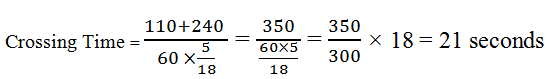New Students Offer - Use Code HELLO

# Problems Based on Trains Made Easy

Today, I am sharing 'Problems based on Trains' chapter. I hope it will be beneficial to our readers.

Introduction
A train is said to have crossed an object (stationary or moving) only when the last coach (end) of the train crosses the said object completely. It implies that the total length of the train has crossed the total length of the object.

In case of Train, The Distance covered by the Train = Length of Train + Length of Object

You have to keep in mind some Formulae which are given below:### Conversion of  km/hr into meter/sec

Following formula is used in this case### Conversion of meter/sec into km/h

The formula is:We can find the basic formula for the time required for a train to cross different type of objects.### Different types of Objects

On the basis of various types of objects that a train has to cross, we find the following different cases:

Question
A train 110 meter long travels at 60 km/h. How long does it take to cross,
a) a telegraph post
b) a man running at 6 km/h in the same direction
c) a man running at 6 km/h in the opposite direction
d) a platform 240 meter long
e) another train 170 meter long standing on another parallel track
f) another train 170 meter long, running at 54 km/h in same direction
g) another train 170 meter long, running at 80 km/h in opposite direction

Solution
a) We have to convert the speed of train from km per hour to meter per second by applying following formula:
Speed of Train = 60 km/h × 5/18 m/sec
The telegraph post is a stationary object with negligible length  so following formula will be applied:
t = Length of Train/Speed of Train
Crossing Time =b) The man is running in the same direction that means object is moving but of negligible length. Hence, formula will be:
Time = Length of Train / Speed of Train - Speed of Man
Crossing Time =c) The man is running in the opposite direction so following formula will be applied:
Crossing Time = Length of Train / Speed of Train + Speed of Man
Crossing Time =d) The platform is stationary but of some length so, following formula will be applied:
Crossing Time = Length of Train + Length of Platform / Speed of Traine) Another Train is standing (stationary), so following formula will be applied:
Crossing Time = Length of First Train + Length of Second (Stationary) Train / Speed of Trainf) Another train is running in the same direction, then following formula will be applied:
Crossing Time = (Length of First Train + Length of Second Train) / (Speed of First Train - Speed of Second Train)
Crossing Time =168 Seconds = 2 minutes 48 seconds

g) Another train is running in opposite direction so following formula will be applied
Crossing Time = Length of First Train + Length of Second Train / Speed of### Tricky Question

You have to learn the basic formulae only then you can easily solve any question from this chapter.
Question
A train passes a platform in 36 seconds and a man standing on the platform in 20 seconds. If the speed of the train is 54 km/h, find the length of the platform.
Solution
Speed = Distance /Time
Because Time is given in seconds so we have to convert the speed into meter/second.
Speed (Velocity) = 54 × 5/18 = 15 meter per second